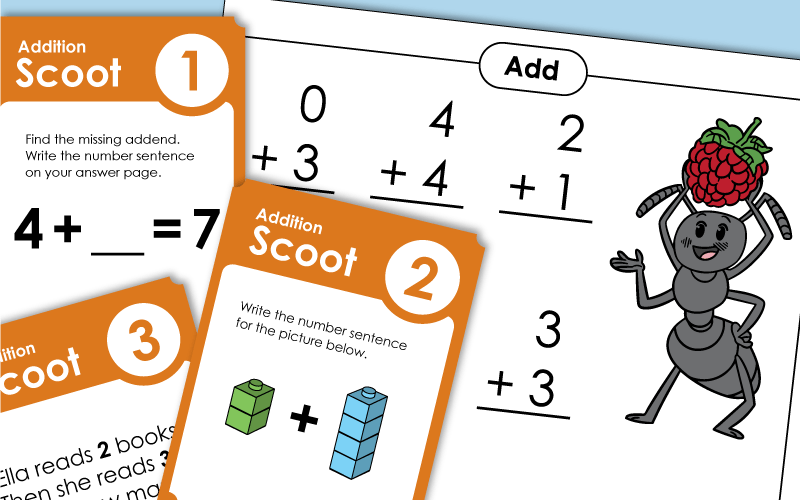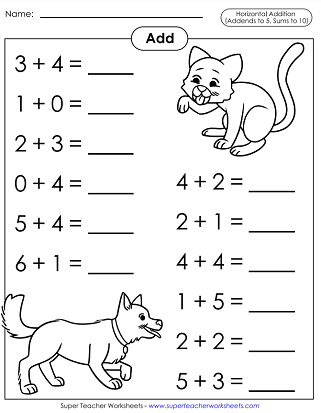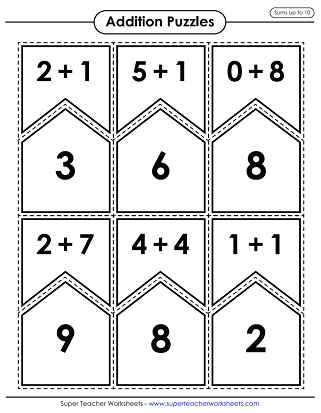# Basic Addition (With Sums up to 10)

These are kindergarten-level addition activities. These worksheets align with Common Core Standard K.OA.2.Cut out these 36 cards. Flip all the cards face down. Have students take turns flipping the cards to make a match. Example: the "3 + 5" card will match the "8" card.
Students love playing our Scoot! games. There are 30 task cards, answer sheets for 20, 25, and 30 questions, and teacher instructions. This version of Scoot! has simple math addition facts to be solved, ideal for students in grades K - 2.
This printable has a set of 30 bingo boards and 42 calling cards with basic addition facts to call out.
Cut apart the stars into 30 pieces. Have your students combine matching sums and numbers together to form stars.
Use scissors to cut these 36 cards out along the dotted lines. Match the basic addition problem to the matching domino card.
Roll a pair of dice and add the results. This game can be played with standard 1-6 number cubes or 0-5 number cubes.
Players take turns rolling pairs of dice. They add the numbers together to find the sum. Player with the highest sum wins the round.
This is a 2-player game for students to compete and practice their addition facts with each other.
Use scissors to cut along the dotted lines and separate all of the puzzle pieces. On the attached page, there are several different ways for your students to play and learn with these puzzles.

This worksheet has pictures of red and white counters. Add to find the sums.
Draw a picture to solve each of these four word problems. Then write a number sentence for each.
This printable worksheet provides number lines to help students solve the addition problems.
Use the dominoes to make and solve these basic addition problems.

Help the butterfly find the flowers. Along the way, answer the addition facts with sums to ten.
Cut out the numbers (sums) on the bottom of the page. Match each sum with the correct addition fact. Glue the sums in the correct boxes.
Write the answers to the addition problems on the caterpillar. All addends are 5 or less. All sums are 10 or less.
Students will use their knowledge of basic math facts to balance the scales on this worksheet. For example: 4 and 3 on one side of the scale will equal 7 on the other side of the scale.

This printable worksheet has basic addition problems to solve. Next, use the sums to determine what color to use for each space. An airplane will appear in the finished picture.
Solve the basic math problems in the picture. Use the answers to find what color each space should be and reveal a beach scene.
Solve basic addition problems and color the areas based on the sum. The picture will reveal a cute fox.
This printable worksheet is a fun way for students to practice their basic addition facts. Solve the addition problems and color the space based on the sum to reveal a robot.
Solve the basic math facts on this worksheet and color each section according to the key at the bottom. The completed worksheet will reveal a rocket ship in space.
Students will solve the basic addition problems and color in the spaces to reveal school supplies and an apple on a desk.
Solve the basic math facts on this page and then color by number to reveal a colorful sailboat.
Solve and color in this mystery picture according to the key to reveal a sunny beach with colorful seashells.
These two mystery picture worksheets look quite similar, but depending on which one you complete, you'll get a different picture!
Solve the addition problems and color according to the key to see which of the two mystery pictures each worksheet reveals.

## Math Fact Practice

Write the answers to nine addition facts and color the pictures. Addends are 5 or less. Sums are also 5 or less. (examples: 2+3=5 and 0+3=3) Features illustrations of kids dressed as superheroes.
This page has nine basic facts on it. All facts have addends of 5 or less and sums of 10 or less. (examples: 4+4=8 and 3+3=6) Includes cute, colorable illustrations of ants carrying food.
Color and solve the math problems. Addends are 5 or less. Sums are 10 or less. (examples: 3+4=7 and 4+4=8)
Features illustrations of a cat and a pig in a swimming pool.
Solve basic addition facts laid out horizontally. There are 12 addition problems, addends to 5 and sums to 10.
Each of these facts has addends below 10 and sums of 10 or less. Illustrations show an astronaut and planets.
The problems on this page has addends and sums of 10 or less. (examples: 8+2=10 and 5+4=9)

This worksheet has ten-frames with red and white counters all adding up to ten. Students will find the missing number to complete the math fact.
On this worksheet, students will use the number sentence to draw the counters in the ten-frame and find the sum.
This printable uses ten-frames to help students find the right number sentence and use their addition skills to determine the sum of each math fact.
The first part of this worksheet has students using the counters in the ten-frame to find the number sentence. The second part uses the number sentence to fill in the ten-frame.
Read each word problem to fill out the ten-frame, write out the math fact, and find them sum.
Use scissors to cut out the ten-frame boxes on the bottom of the worksheet and glue them to the matching number sentence above.
This mini-book is a fun way for students to practice with ten-frames and addition skills. Cut out the pages and assemble, then fill out the ten-frames and solve the math fact.
This printable has 15 pennants that can be cut out and colored. Then solve the ten-frame addition fact. Lastly, these pennants can be strung up on a rope or string to hang in the classroom.

## Flashcards

This file contains flashcards for reviewing basic addition facts with sums to 10. There are 9 cards per page. The final page has a sorting mat.

This is a simple addition table that students can use for reference. Sums on the vertical and horizontal axis go up to 5.

These basic addition fact worksheets are slightly more difficult, for students in grades 1 and 2. All problems have sums less than 20.

We have many more basic addition worksheets. Daily word problems, doubles, doubles +1, making 10, and much more. Visit this link for a full list of basic addition topics.

## Sample Worksheet ImagesMy Account
Site Information